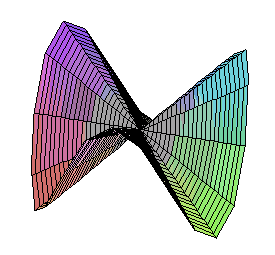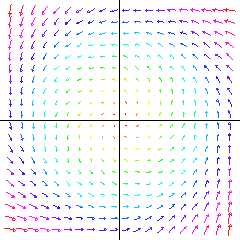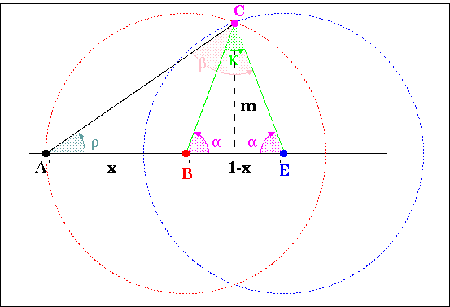## 2000-Level Mathematics Courses at Trent University (2009-2010)

Notes:

 1000-Level ....... 2000-Level ....... 3000-Level ....... 4000-Level
Math. 200 Calculus in several dimensions Discontinued

This course has been replaced with MATH 2110H[201H] and MATH 2120H[202H]

Math. 206H Analysis I: Introduction to Analysis Discontinued

This course has been renamed as MATH 3790H[309H]

Math. 207H Introduction to numerical & computational methods Discontinued

This course has been renamed as MATH-COIS 2180H[203H]

MATH 2080 Mathematics for the Contemporary Classroom Fall-Winter 2009-2010

A course in mathematics and mathematical thinking for prospective school teachers. Number systems and counting, graphs and networks, probability and statistics, measurement and growth, symmetry, computers and mathematics. Not available to students enrolled in or with credit for any of Math 1100 , MATH 1350H[135H] or MATH-COIS 2600H[260H] or their equivalents. Not for credit towards any major in Mathematics.

• Note: Instructor's approval required; enrolment limited.
• Meetings: Three-hour lecture/workshop weekly.
• Timetables
• Section A: Full Year
• Section B: Not offered
• Section C: Not offered
• Instructor:
• Section A:   Gary Zuliani
• Section B:   Not offered
• Section C:   Not offered

MATH 2084H[284H] Recreational Mathematics Not offered 2009-2010

Description: This is a general-interest course about topics in mathematics that are studied for fun. These topics include magic squares, logic puzzles, toys and tricks with mathematical content, polygonal dissections and tiling problems, etc. These topics will be especially interesting for anyone who wants to become a math teacher, and will also appeal to non-mathematicians who have a casual interest in math (e.g. geometric puzzle aficionados, readers of Martin Gardner's ``Mathematical Games'' and similar columns in Scientific American, etc.). Some possible topics include:

1. Magic squares
• History.
• Algorithms for generating magic squares.
• Tricks for modifying magic squares to create new ones.
• Magic squares with additional properties.
2. Logic problems and puzzles
• Deductions involving logical alternatives.
• Puzzles and paradoxes involving self-references, including:
• The lady or the tiger.
• The unexpected hanging.
3. Dissections
• Cutting up polygons into pieces and reassembling them into other polygons.
• When can a polygon be dissected into another polygon?
• What happens with non-polygonal shapes?
• What happens if pieces are ``cut'' in more complex ways?
4. Tilings
• What combinations of tiles can be used to cover a floor?
• Periodic and non-periodic tilings.
• Connections between dissections and tilings.
5. Polyominoes
• What shapes can be created with given sets of polyominoes?
• Tilings using polyominoes.
• Reptiles (polyominoes which can be tiled by smaller copies of themselves).
• Extensions of polyominoes to three dimensions, e.g. the Soma cube.
6. Map colouring
• The general Four-Colour Theorem.
• The Two-Colour Theorem for a map created by drawing (possibly overlapping) circles. Investigate how far this theorem can be extended.
7. Arithmetic tricks
• Puzzles based on tables for arithmetic operations.
• Divisibility tests and why they work.
8. Conway's Game of Life
• Stable, periodic, and moving patterns in the Game of Life.
• ``Garden of Eden'' patterns.
• Computing using the Game of Life and other cellular automata.
9. Arrangements
• Kirkman Schoolgirl Problem and Steiner systems.
• Small finite affine and projective geometries.
• Applications to puzzles, experimental design, and coding.
• Prerequisite: Any Grade 12 Mathematics credit or equivalent; and two full credits in any subject(s) at the 100 level, or permission of the instructor.
• Not for credit towards a major in mathematics.

• Meetings: Three lectures and one tutorial weekly.
• Not offered Class timetable
• .
• Instructors:   Not offered.

MATH 2085H[285H] The Mathematics of Art, Architecture and Music Not offered 2009-2010

Description: This is a general interest, interdisciplinary course exploring the interactions between mathematics and the arts. Its goal is to introduce students (with or without prior mathematical experience) to the beauty of mathematics, and show how mathematical concepts can help elucidate aesthetic principles. Possible topics include:

1. Symmetry:
• The 5 regular (`Platonic') polyhedra and 13 semiregular (`Archimedean') polyhedra.
• Frieze groups, with applications to canons and fugues (especially J.S. Bach).
• Planar tilings and crystallographic groups. Medieval Islamic art and the tilings of M.C. Escher.
2. Self-similarity and Fractals:
• Fractal geometry in natural forms (e.g. trees, snowflakes, mountains, coastlines).
• Fractals and multiscale self-similarity in art (e.g. 19th century Japanese woodcuts) and music (e.g. Balinese Gamelan music and Colin McPhee, or J.S. Bach's canons).
3. The golden ratio: Fibonacci numbers, golden rectangles, golden spirals, importance in classical and Renaissance art.
4. Musical Harmony:
• Musical notes as frequencies; an octave as a frequency doubling. Harmony as low-denominator frequency ratios.
• The circle of fifths
• Harmonics and chords.
• Well-tempered tuning and base-2 logarithms.
5. Modular arithmetic as a model of cyclical time. Applications to musical rhythm patterns.
6. Self-reference:
• Induction and recursion in mathematics.
• Self-referential structures and metamathematics. Gödel's Incompleteness Theorem.
• Self-reference and postmodernism in art and literature.
7. Mathematics in architecture: the Parthenon; Renaissance military engineering; mazes and labyrinths.
8. Art inspired by mathematics. For example:
• Mathematically themed visual art and sculpture (e.g. M.C. Escher, Sol Lewitt).
• Mathematically inspired music (e.g. Iannis Xenakis).
• Mathematically inspired literature. For example:
• Rudy Rucker's White light.
• Douglas Hofstadter's Gödel, Escher, Bach.
• Michael Crichton's Jurassic Park.
• The short fiction of Jorge Luis Borges, e.g. ``The Library of Babel'' or ``The Immortal''.
• The short ficton of Greg Egan, e.g. ``Wang's Carpets'' or ``Unstable Orbits in the Space of Lies''.
• Alex Kasman's Reality Conditions.
• William Frucht's Imaginary Numbers.
• Mathematics in drama (e.g. David Auburn's Proof, Tom Stoppard's Arcadia, Michael Frayn's Copenhagen, John Mighton).
• Mathematics in television and cinema (e.g. Pi and Numb3rs).
• Prerequisite: Any Grade 12 Mathematics credit or equivalent; and two full credits in any subject(s) at the 100 level, or permission of the instructor.

Recommended pre/corequisites: MATH 2260H[226H] , or CUST 211 (Drawing) or CUST 216 ( Introduction to Visual Studies) or CUST-ENGL 229 (Science Fiction) or CUST 245 (Music and Society).

These recommendations are for student interest only; they are not required.

• Not for credit towards a major in mathematics.

• Meetings: Three lectures and one tutorial weekly.
• Not offered Class timetable.
• Instructors:   Not offered

MATH 2110H[201H] Calculus of Several Variables Fall 2009The Monkey's Saddle is not differentiable at zero, but all tangent lines exist there. Multivariable functions, curves and surfaces in two and three dimensions. Partial differentiation and applications. Multiple integrals. Prerequisite: MATH 1100 . Pre/Co-requisite:Grade 12U Algebra, Geometry and Discrete Mathematics or (highly recommended) MATH 1350H[135H] .. Class timetable Instructor: Reem Yassawi
MATH 2120H[202H] Vector Calculus Winter 2010

Parametric curves and surfaces, vector functions and fields. Line integrals, Green's Theorem. Surface integrals, curl and divergence, Stokes' and Divergence Theorems.

MATH-PHYS 2150H[205H] Ordinary Differential Equations Fall 2009
 Prerequisite: MATH 1100 . Corequisite: MATH 2110H[201H] . Recommended: MATH 1350H[135H] . Meetings: Three lectures and one tutorial weekly. Class timetable Instructor: Wenying Feng Synopsis::  Analytical and graphical solutions are studied for first-order, higher-order and systems of first-order equations. Applications are shown by linear and nonlinear Mathematical models in Physics, Biology and other areas. Laplace transform and its applications are also introduced. (Time permitting, power series solutions and/or numerical methods may also be covered.)A two-dimensional vector field determines a flow in the plane.

Overview: Ordinary differential equations (ODEs) model physical systems which evolve continuously in time. For example, suppose the state of the system is described by a single variable $x\left(t\right)$, and satisfies the equation:
 d x(t) ----- = -x(t). d t
If $x\left(0\right)=5$, then the unique solution to this equation is the curve $x\left(t\right) = 5 e-t$. This curve starts at $5$, and asymptotically approaches $0$. We say that $0$ is an equilibrium state for the system.

Suppose the state of the system at time $t$ is given by a real vector x(t) in RN. Then an ODE for this system has the form:
 d x ----- = V[x(t)]. d t
This says that the velocity of the system (namely $d$x/dt) is a determined entirely by it's state (namely x), via some function $V:$RN ---->RN.

`     `

ODEs are ubiquitous in applied mathematics. For example:

• In physics: ODEs model trajectories in classical and relativistic mechanics.
• In chemistry: ODEs describe the reaction kinetics of chemical systems.
• In biology: ODEs describe the evolving populations of interacting species, fluctuating endocrine levels in the body, or neural activity in the brain.
• In economics: ODEs describe business cycles.

Given an ODE, we can ask four questions:

1. Do solutions exist? In other words, given an initial state x0, is there a smooth curve x(t) satisfying x(0)=x0 and the ODE?
2. Is this solution unique?
3. What is an explicit formula describing the solution?
4. What is the long-term qualitative behaviour of the system?

MATH-COIS 2180H[203H] Introduction to numerical & computational methods
(formerly Math 207H)
Winter 2010

Error analysis, non-linear equations, linear systems, interpolation methods, numerical differentiation and integration and initial value problems.

MATH 2200H[220H] Mathematical Reasoning Fall 2009

This course is intended for mathematics majors in the first or second year of their program, and introduces the essential concepts, methods, paradigms and abstractions of higher mathematics. It is recommended as a pre/corequisite for upper level pure math courses such as ```MATH 3720H[302H] , 307H, 309H, 310H, 320H, 321H, 332H, 336H, 407H, 409H, 410H, 415H, 431H, 432H, 433H, 435H, 437H, 416H, 461H, 462H, 471H, and 472H```. We will cover most of the following topics:

• Proofs and logic: Modus ponens; proof by cases; proof by contradiction (eg: Euclid's proof that there are infinitely many prime numbers; Cantor's diagonal argument). Proof by induction (e.g. the Euclidean algorithm; Lamé's theorem.)
• Sets and functions: Sets and subsets; union and intersection; de Morgan's laws. Functions: injective, surjective, and bijective. Inverse images.
• Combinatorics: Counting arguments; the Pigeonhole Principle; factorials, binomial coefficients, Pascal's formula; the binomial theorem. Multinomial coefficients and the multinomial theorem.
• Transfinite Arithmetic: Cantor's definition of cardinality; The sets N, Z, N2, and Q all have cardinality Aleph0. Cantor's diagonal argument, showing that R has cardinality c > Aleph0.
• Complex numbers: The complex plane in Cartesian and polar coordinates. Addition, multiplication, and the exponential map, and their geometric interpretations. de Moivre's formulae.
• Basic Number Theory:
• Divisibility; greatest common divisors, lowest common multiples. The Euclidean algorithm. Fibonacci numbers and Lamé's theorem.
• Linear diophantine equations. Brahmagupta's theorem.
• Prime numbers and prime factorization. The Fundamental Theorem of Arithmetic.
• Modular arithmetic: Modular addition and multiplication; the concept of equivalence class, with congruence classes as the prototypical example. Linear congruence equations. Fermat's little theorem.
• Possible additional topics: We may have time for one or two of the following topics:
• Symmetry and Transformation groups: Symmetries of plane figures. Transformation groups. Examples: dihedral group, matrix groups, permutation groups. Subgroups, group homomorphisms and group isomorphisms.
• Introduction to topology: Curves, surfaces, and manifolds. Homeomorphism and homotopy. The Euler-Poincaré invariant and the fundamental group.

MATH 2260H[226H] Geometry I: Euclidean geometry Winter 2010

Geometry is the oldest part of mathematics; its origins are lost in antiquity. Classical Euclidean geometry applies logical deduction to discover the relationships of lines, points, and circles in the plane. For more than two thousand years, it has been the ideal of clarity and elegance to which all other mathematics aspires. In this course, we will cover some subset of the following topics:

 Constructions using compass & straight-edge. Nonconstructability. Triangles: Similarity & congruence. Ceva's theorem and corollaries. Symmetry: Isometries; Kleinian geometry. Symmetric planar figures. Friezes, tilings, and crystal structures. Symmetric polyhedra (Platonic & Archimedean). Isaac Newton's geometric development of calculus in the Principia. (time permitting) Introduction to non-Euclidean geometry: The Parallel Postulate. Spherical geometry. Hyperbolic geometry. Toroidal geometry.A construction of a pentagon using only compass and straightedge. The angle $\alpha$ is exactly one fifth of a full circle. [Courtesy of Adam Hulcoop]

Recommended for Education students.

• Prerequisite: Either MATH 1005H[105H] or MATH 1100 (with OAC Algebra/Geometry recommended), or 135H.
• Meetings: Two lectures and one tutorial weekly.
• Class timetable
• Instructor:  David Poole

MATH 2350H[235H] Linear Algebra II: Vector Spaces Winter 2010

Vector spaces, basis and dimension, inner product spaces, orthogonality, linear transformations, diagonalization, determinants, eigenvalues, quadratic forms, least squares, the singular value decomposition.

MATH 2560H[256H] Introduction to Statistical Inference
(formerly the first half of Math 355)
Fall 2009

Introduction to mathematical statistics: Methods of point estimation, confidence intervals, hypotheses testing, comparative inferences, nonparametric methods.

• Prerequisite: MATH 1550H[155H] . Also ssumes a background in probability and calculus.
• Meetings: Three hours lecture and one hour tutorial weekly.
• Class timetable
• Instructor:   Michelle Boue

Math.-Comp. Sci. 2600H Discrete Structures Fall 2009

Mathematics related to computer science including propositional logic, recursive functions, combinatorics, graphs and networks, Boolean algebras. Applications to languages, analysis of algorithms, optimization problems, coding theory, and circuit design.

• Prerequisite: MATH 1100 or MATH 1350H[135H] ; or COIS 102H together with one Grade 12 U or U/C or OAC credit in Mathematics.
• Recommended: OAC Finite Mathematics.
• Meetings: Three hours weekly.
• Class timetable
• Instructor:   Bing Zhou